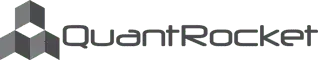Disclaimer

# Researching the Momentum Factor¶

Momentum investing says that excess returns can be generated by buying recent winners and selling recent losers. In this notebook we will research the momentum factor on our universe of demo stocks. This will help us determine whether we have a profitable idea before turning to a full backtest.

Next, we use closing prices to calculate our momentum factor. We calculate momentum using a twelve-month window but excluding the most recent month, as commonly recommended by academic papers.

Now that we have the twelve-month returns, we calculate the next day returns:

To see if the twelve-month returns predict next-day returns, we will split the twelve-month returns into bins and look at the mean next-day return of each bin. To do this, we first need to stack our wide-form DataFrames into Series.

Use pandas' qcut function to create the bins:

Now group the next day returns by momentum bin and plot the mean return:

For a predictive factor, the higher quantiles should perform better than the lower quantiles.

## Next Up¶

Part 4: Moonshot Strategy Code